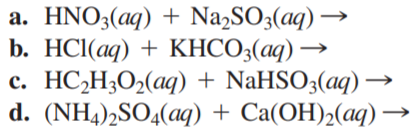×
Get Full Access to Chemistry: A Molecular Approach - 3 Edition - Chapter 4 - Problem 90e
Get Full Access to Chemistry: A Molecular Approach - 3 Edition - Chapter 4 - Problem 90e

×

# Complete and balance each gas-evolution equation.ISBN: 9780321809247 1

## Solution for problem 90E Chapter 4

Chemistry: A Molecular Approach | 3rd Edition

• Textbook Solutions
• 2901 Step-by-step solutions solved by professors and subject experts
• Get 24/7 help from StudySoup virtual teaching assistantsChemistry: A Molecular Approach | 3rd Edition

4 5 1 326 Reviews
27
1
Problem 90E

Complete and balance each gas-evolution equation.Step-by-Step Solution:
Step 1 of 3

Solution: Here, we are going to calculate the percentage by mass of calcium carbonate in the given sample. Step1: Given, the volume of NaOH solution used = 11.56 mL = 11.56 / 1000 L = 0.01156 L The molarity of NaOH solution used = 1.010 M Therefore, number of moles of NaOH solution = Volume of solution in litres X Molarity = 0.01156 L X 1.010 M = 0.012 mol Step2: Again, the volume of HCl solution used = 30 mL = 30 / 1000 L = 0.030 L The molarity of HCl solution used = 1.035 M Therefore, number of moles of HCl solution = Volume of solution in litres X Molarity = 0.030 L X 1.035 M = 0.031 mol Since, 1 mole of NaOH will react with 1 mole of HCl for complete neutralisation, therefore, 0.012 mol of NaOH will react will 0.012 mol of HCl for neutralization. Thus, number of moles of HCl reacted with limestone = total number of moles of HCl - number of moles of HCl that reacted with NaOH = (0.031 - 0.012) mol = 0.019 mol Step3: The reaction between HCl and CaCO takes pla3 in the following way: 2HCl(aq) + CaCO (s) 3-------> CaCl (aq) 2H CO (a2 3 From the above equation it is clear that, 2 moles of HCl reacts with 1 mole of CaCO for 3 complete neutralisation. Therefore, 0.019 mol of HCl will react with (0.019 / 2 = 0.0095) mol of CaCO for complete 3 neutralisation. Step4: Now, number of moles of CaCO = mass of3aCO / molar mass 3 Therefore, mass of CaCO used =3umber of moles X molar mass = 0.0095 X 100.1 g [molar mass of CaCO = 100.1 g/mol] 3 = 0.95 g Thus mass percentage of CaCO = (mass of CaCO used / mass of limestone) X 100 3 3 = (0.95 / 1.248) X 100 % = 0.761 X 100 % = 76.1 % -------------------

Step 2 of 3

Step 3 of 3

##### ISBN: 9780321809247

The full step-by-step solution to problem: 90E from chapter: 4 was answered by , our top Chemistry solution expert on 02/22/17, 04:35PM. Since the solution to 90E from 4 chapter was answered, more than 408 students have viewed the full step-by-step answer. The answer to “?Complete and balance each gas-evolution equation.” is broken down into a number of easy to follow steps, and 6 words. Chemistry: A Molecular Approach was written by and is associated to the ISBN: 9780321809247. This full solution covers the following key subjects: HCI, solution, Rock, neutralization, calculate. This expansive textbook survival guide covers 82 chapters, and 9454 solutions. This textbook survival guide was created for the textbook: Chemistry: A Molecular Approach, edition: 3.

Unlock Textbook Solution